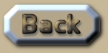Simulation of Earth's orbit under Relativity

 Relativity and the orbit of the Earth A tricky thing about computing the orbit of the Earth – is that the Moon’s gravity wobbles the Earth by as much as 9282km per month. So to simplify this n-body-problem, the evolutionary algorithm orbit-gravity-sim-12.exe (OGS12) only maps the orbit of the Earth-Moon barycenter around the Sun. The maximum and minimum distances (aphelion and perihelion) of the orbit are thus taken to be a bit closer together than the true amounts of the Earth itself. The mass contained by this orbit is then also the sum of both the Earth and the Moon. Scenario  of the OGS12 algorithm shows the natural Newtonian orbit with an error-margin of about +-0.026km per orbit averaging to +-0.0003km (about 1 foot!) per orbit over 16 orbits. This error-margin is due to the orbit being a polygon of about 21000 sides. I will examine detail as to how this occurs later in the chapter. Scenario  shows that the Earth’s orbit will be influenced by both Special Relativity and General Relativity to the extent of an outwards drift of a bit more than 1km per orbit: .
 So gravity-velocity from General Relativity has a larger impact on this orbit than does a loss in velocity from Special Relativity (unlike Mercury). There is uncertainty as to what the perihelion and aphelion of the barycenter actually are because all the websites looked at do not account for the change that the Moon causes to the Earth’s position. If we take the maximum to be at New Moon it could be 4641km more than the widely quoted typical answer of 152 098 232km; alternatively that amount could be the maximum including the 4641 caused by the Earth’s wobble at New Moon. So I have taken the claim to the maximum distance quite literally. But it matters little as a matter of principle because the changes made by the Relativities are proportional to those amounts. .This is an extract summary of Chapter XXX of the book: Flight Light and Spin